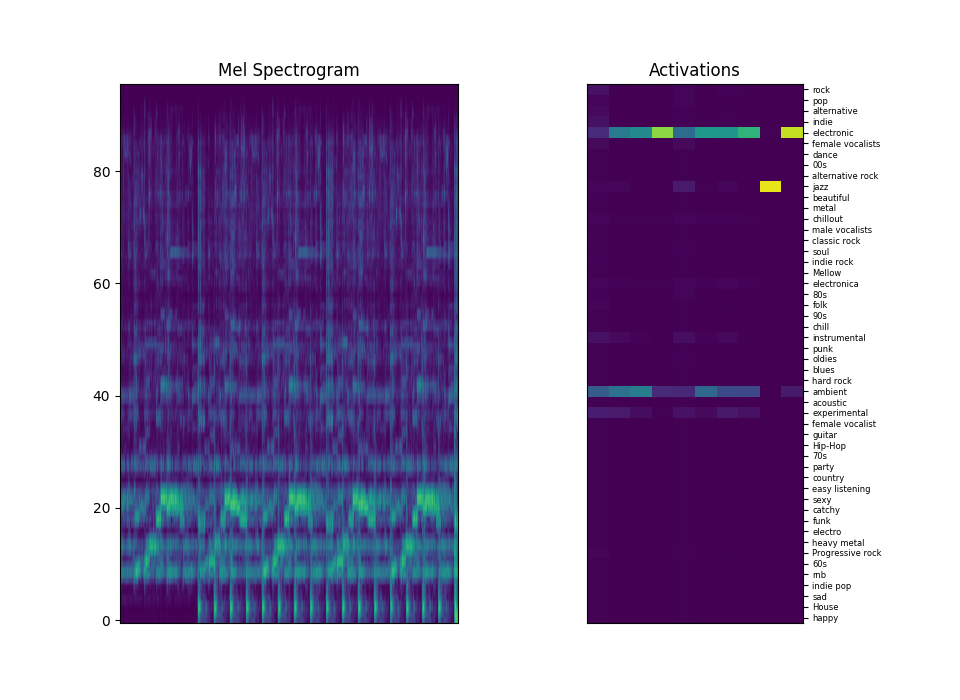# Real-time music auto-tagging¶

In this tutorial, we use Essentia’s TensorFlow integration to perform auto-tagging in real-time. Additionally, this serves as an example of TensorFlow inference in streaming mode and can be easily adapted to work offline.

## Setup¶

To install Essentia with TensorFlow support, refer to the Setup section of our previous Music auto-tagging, classification, and embedding extraction tutorial for instructions.

Additionally, we rely on the `pysoundcard` package to capture the audio loopback of the system and feed Essentia in real-time. This way we can easily test our models with any music coming from our local player or browser.

```!pip -q install pysoundcard
```

Let’s download `MusiCNN`, one of our auto-tagging models. This and more models are available from the Essentia models’ site.

```!wget -q https://essentia.upf.edu/models/autotagging/msd/msd-musicnn-1.pb
!wget -q https://essentia.upf.edu/models/autotagging/msd/msd-musicnn-1.json
```

Then we import the required packages and Essentia algorithms. In this case, we use the TensorFlow functionalities in streaming mode.

```import json

from essentia.streaming import (
VectorInput,
FrameCutter,
TensorflowInputMusiCNN,
VectorRealToTensor,
TensorToPool,
TensorflowPredict,
PoolToTensor,
TensorToVectorReal
)
from essentia import Pool, run, reset
from IPython import display
import numpy as np
import matplotlib.pyplot as plt
from scipy.special import softmax
import soundcard as sc

%matplotlib nbagg
```

Define the analysis parameters. To make this demo work in real-time, we tweaked some of the analysis parameters of `MusiCNN`. While it was trained on patches of size 187 (~3 seconds) we set `patch_size` to 64 (~1 second) to increase the prediction rate. You can experiment with the `patch_size` and `display_size` parameters to modify the prediction rate to your taste.

```with open('msd-musicnn-1.json', 'r') as json_file:

model_file = 'msd-musicnn-1.pb'
n_classes = len(classes)

# Analysis parameters.
sample_rate = 16000
frame_size = 512
hop_size = 256
n_bands = 96
patch_size = 64
display_size = 10

buffer_size = patch_size * hop_size
```

Instantiate the algorithms. With this, we create a network similar to the one used inside `TensorflowPredictMusiCNN`, the wrapper algorithm presented in the previous tutorial. However, by instantiating the algorithms separately we gain additional control required for real-time usage.

```buffer = np.zeros(buffer_size, dtype='float32')
vimp = VectorInput(buffer)
fc = FrameCutter(frameSize=frame_size, hopSize=hop_size)
tim = TensorflowInputMusiCNN()
vtt = VectorRealToTensor(shape=[1, 1, patch_size, n_bands],
ttp = TensorToPool(namespace=input_layer)
tfp = TensorflowPredict(graphFilename=model_file,
inputs=[input_layer],
outputs=[output_layer])
ptt = PoolToTensor(namespace=output_layer)
ttv = TensorToVectorReal()
pool = Pool()
```

Connect the algorithms. We also store the mel-spectrograms in the `Pool` for visualization purposes.

```vimp.data   >> fc.signal
fc.frame    >> tim.frame
tim.bands   >> vtt.frame
tim.bands   >> (pool, 'melbands')
vtt.tensor  >> ttp.tensor
ttp.pool    >> tfp.poolIn
tfp.poolOut >> ptt.pool
ptt.tensor  >> ttv.tensor
ttv.frame   >> (pool, output_layer)
```

Create a callback function that will be called every time the audio buffer is ready to process.

```def callback(data):
buffer[:] = data.flatten()

# Generate predictions.
reset(vimp)
run(vimp)

# Update the mel-spectrograms and activations buffers.
mel_buffer[:] = np.roll(mel_buffer, -patch_size)
mel_buffer[:, -patch_size:] = pool['melbands'][-patch_size:, :].T
img_mel.set_data(mel_buffer)

act_buffer[:] = np.roll(act_buffer, -1)
act_buffer[:, -1] = softmax(20 * pool[output_layer][-1, :].T)
img_act.set_data(act_buffer)

f.canvas.draw()
```

Initialize the plots and start processing the loopback stream.

```mel_buffer = np.zeros([n_bands, patch_size * display_size])
act_buffer = np.zeros([n_classes, display_size])

pool.clear()

f, ax = plt.subplots(1, 2, figsize=[9.6, 7])
f.canvas.draw()

ax.set_title('Mel Spectrogram')
img_mel = ax.imshow(mel_buffer, aspect='auto',
origin='lower', vmin=0, vmax=6)
ax.set_xticks([])

ax.set_title('Activations')
img_act = ax.matshow(act_buffer, aspect='0.5', vmin=0, vmax=1)
ax.set_xticks([])
ax.yaxis.set_ticks_position('right')
plt.yticks(np.arange(n_classes), classes, fontsize=6)

# Capture and process the speakers loopback.
with sc.all_microphones(include_loopback=True).recorder(samplerate=sample_rate) as mic:
while True:
callback(mic.record(numframes=buffer_size).mean(axis=1))
```
```<IPython.core.display.Javascript object>
```